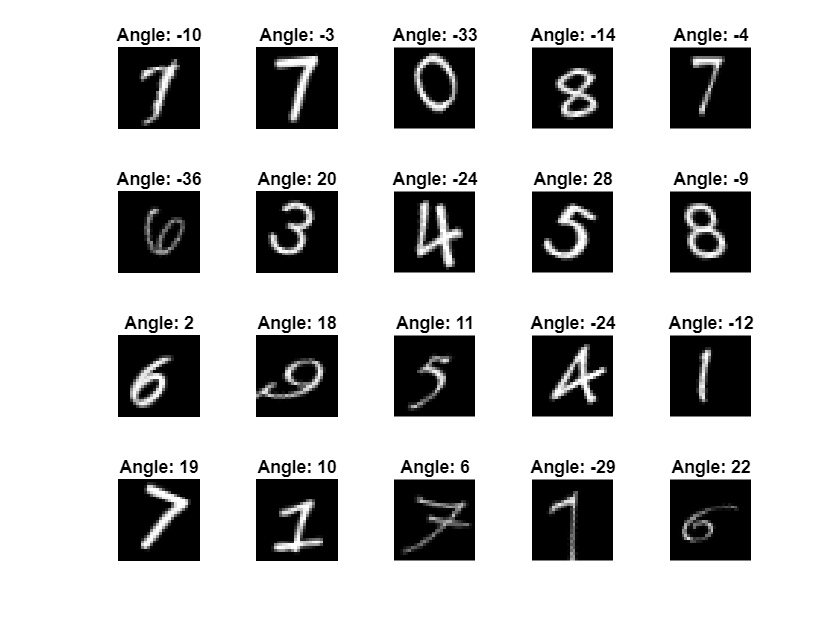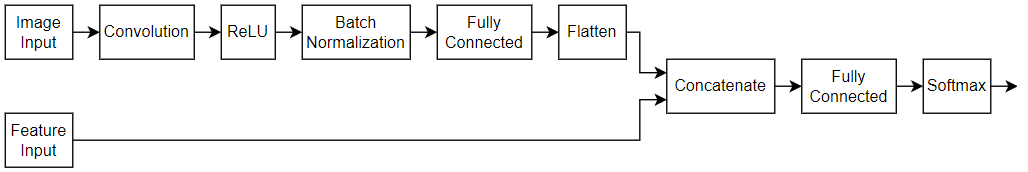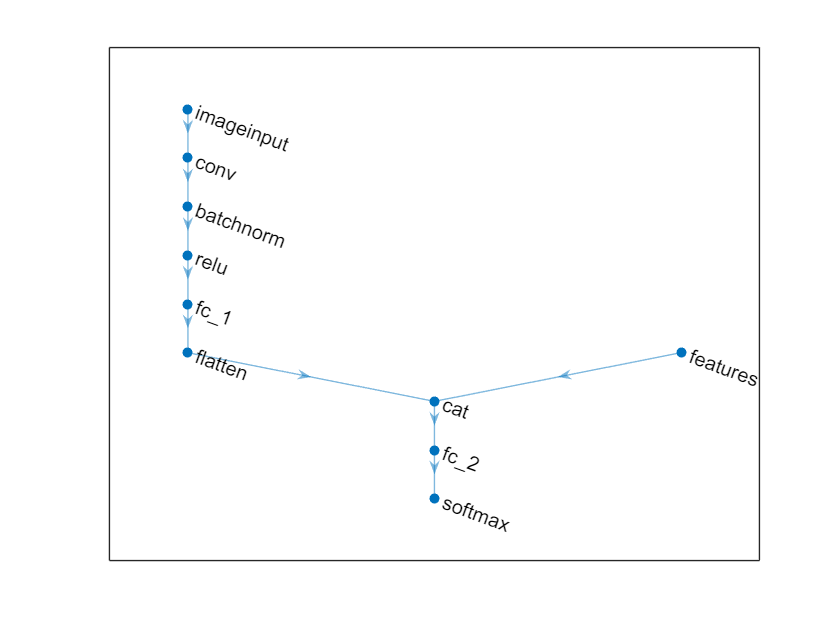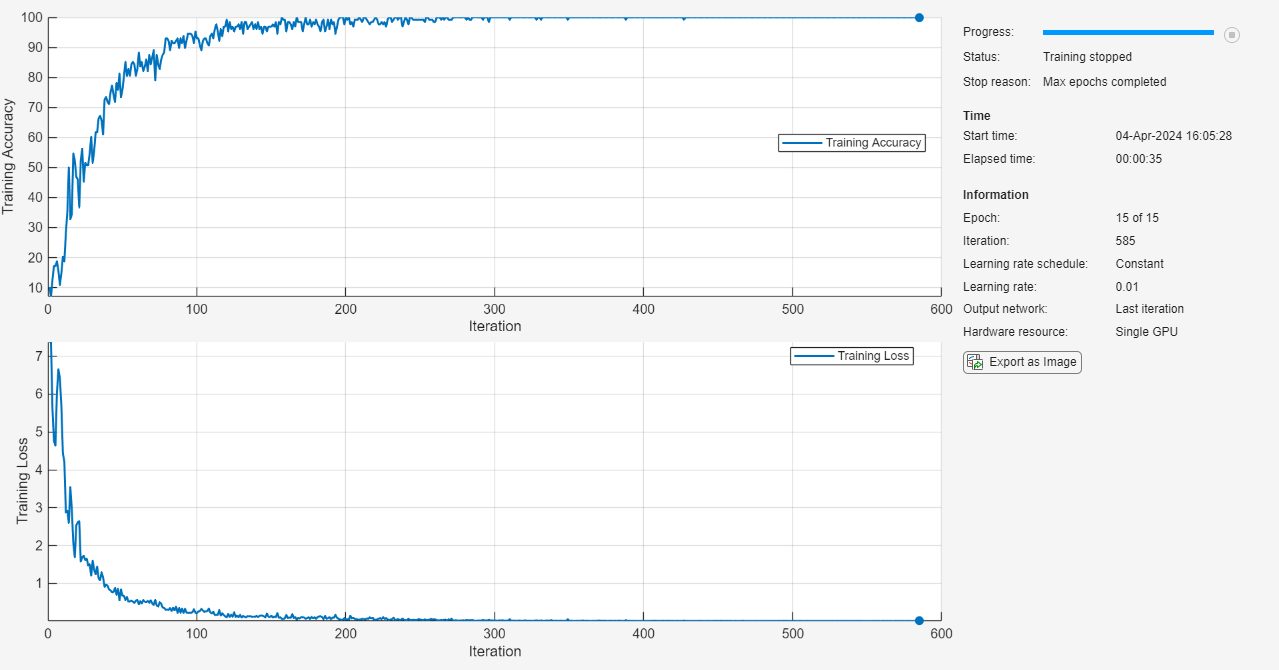# Train Network on Image and Feature Data

This example shows how to train a network that classifies handwritten digits using both image and feature input data.

Load the digits images `XTrain`, labels `YTrain`, and clockwise rotation angles `anglesTrain`. Create an `arrayDatastore` object for the images, labels, and the angles, and then use the `combine` function to make a single datastore that contains all of the training data. Extract the class names and the height, width, number of channels, and number of nondiscrete responses.

```[XTrain,YTrain,anglesTrain] = digitTrain4DArrayData; dsXTrain = arrayDatastore(XTrain,'IterationDimension',4); dsAnglesTrain = arrayDatastore(anglesTrain); dsYTrain = arrayDatastore(YTrain); dsTrain = combine(dsXTrain,dsAnglesTrain,dsYTrain); classes = categories(YTrain); [h,w,c,numObservations] = size(XTrain);```

Display 20 random training images.

```numTrainImages = numel(YTrain); figure idx = randperm(numTrainImages,20); for i = 1:numel(idx) subplot(4,5,i) imshow(XTrain(:,:,:,idx(i))) title("Angle: " + anglesTrain(idx(i))) end```### Define Network

Define the size of the input image, the number of features of each observation, the number of classes, and the size and number of filters of the convolution layer.

```imageInputSize = [h w c]; numFeatures = 1; numClasses = numel(classes); filterSize = 5; numFilters = 16;```

To create a network with two input layers, you must define the network in two parts and join them, for example, by using a concatenation layer.

Define the first part of the network. Define the image classification layers and include a concatenation layer before the last fully connected layer.

```layers = [ imageInputLayer(imageInputSize,'Normalization','none','Name','images') convolution2dLayer(filterSize,numFilters,'Name','conv') reluLayer('Name','relu') fullyConnectedLayer(50,'Name','fc1') concatenationLayer(1,2,'Name','concat') fullyConnectedLayer(numClasses,'Name','fc2') softmaxLayer('Name','softmax')];```

Convert the layers to a layer graph.

`lgraph = layerGraph(layers);`

For the second part of the network, add a feature input layer and connect it to the second input of the concatenation layer.

```featInput = featureInputLayer(numFeatures,'Name','features'); lgraph = addLayers(lgraph, featInput); lgraph = connectLayers(lgraph, 'features', 'concat/in2');```

Visualize the network.

```figure plot(lgraph)```Create a `dlnetwork` object.

`dlnet = dlnetwork(lgraph);`

When using the functions `predict` and `forward` on a `dlnetwork` object, the input arguments must match the order given by the `InputNames` property of the `dlnetwork` object. Inspect the name and order of the input layers.

`dlnet.InputNames`
```ans = 1×2 cell {'images'} {'features'} ```

The `modelGradients` function, listed in the Model Gradients Function section of the example, takes a `dlnetwork` object `dlnet`, a mini-batch of input image data `dlX1`, a mini-batch of input feature data `dlX2,` with corresponding labels `dlY` and returns the gradients of the loss with respect to the learnable parameters in `dlnet`, the network state, and the loss.

### Specify Training Options

Train with a mini-batch size of 128 for 15 epochs.

```numEpochs = 15; miniBatchSize = 128;```

Specify the options for SGDM optimization. Specify an initial learn rate of 0.01 with a decay of 0.01, and momentum of 0.9.

```learnRate = 0.01; decay = 0.01; momentum = 0.9;```

To monitor the training progress, you can plot the training loss after each iteration. Create the variable `plots` that contains `"training-progress"`. If you do not want to plot the training progress, then set this value to `"none"`.

`plots = "training-progress";`

### Train Model

Train the model using a custom training loop. Initialize the velocity parameter for the SGDM solver.

`velocity = [];`

Use `minibatchqueue` to process and manage mini-batches of images during training. For each mini-batch:

• Use the custom mini-batch preprocessing function `preprocessData` (defined at the end of this example) to one-hot encode the class labels.

• By default, the `minibatchqueue` object converts the data to `dlarray` objects with underlying type `single`. Format the images with the dimension labels `'SSCB'` (spatial, spatial, channel, batch), and the angles with the dimension labels `'CB'` (channel, batch). Do not add a format to the class labels.

• Train on a GPU if one is available. By default, the `minibatchqueue` object converts each output to a `gpuArray` if a GPU is available. Using a GPU requires Parallel Computing Toolbox™ and a CUDA® enabled NVIDIA® GPU with compute capability 3.0 or higher.

```mbq = minibatchqueue(dsTrain,... 'MiniBatchSize',miniBatchSize,... 'MiniBatchFcn', @preprocessMiniBatch,... 'MiniBatchFormat',{'SSCB','CB',''});```

For each epoch, shuffle the data and loop over mini-batches of data. At the end of each epoch, display the training progress. For each mini-batch:

• Evaluate the model gradients, state, and loss using `dlfeval` and the `modelGradients` function and update the network state.

• Update the network parameters using the `sgdmupdate` function.

Initialize the training progress plot.

```if plots == "training-progress" figure lineLossTrain = animatedline('Color',[0.85 0.325 0.098]); ylim([0 inf]) xlabel("Iteration") ylabel("Loss") grid on end```

Train the model.

```iteration = 0; start = tic; % Loop over epochs. for epoch = 1:numEpochs % Shuffle data. shuffle(mbq) % Loop over mini-batches. while hasdata(mbq) iteration = iteration + 1; % Read mini-batch of data. [dlX1,dlX2,dlY] = next(mbq); % Evaluate the model gradients, state, and loss using dlfeval and the % modelGradients function and update the network state. [gradients,state,loss] = dlfeval(@modelGradients,dlnet,dlX1,dlX2,dlY); dlnet.State = state; % Update the network parameters using the SGDM optimizer. [dlnet, velocity] = sgdmupdate(dlnet, gradients, velocity, learnRate, momentum); if plots == "training-progress" % Display the training progress. D = duration(0,0,toc(start),'Format','hh:mm:ss'); %completionPercentage = round(iteration/numIterations*100,0); title("Epoch: " + epoch + ", Elapsed: " + string(D)); addpoints(lineLossTrain,iteration,double(gather(extractdata(loss)))) drawnow end end end```### Test Model

Test the classification accuracy of the model by comparing the predictions on a test set with the true labels. Test the classification accuracy of the model by comparing the predictions on a test set with the true labels and angles. Manage the test data set using a `minibatchqueue` object with the same setting as the training data.

```[XTest,YTest,anglesTest] = digitTest4DArrayData; dsXTest = arrayDatastore(XTest,'IterationDimension',4); dsAnglesTest = arrayDatastore(anglesTest); dsYTest = arrayDatastore(YTest); dsTest = combine(dsXTest,dsAnglesTest,dsYTest); mbqTest = minibatchqueue(dsTest,... 'MiniBatchSize',miniBatchSize,... 'MiniBatchFcn', @preprocessMiniBatch,... 'MiniBatchFormat',{'SSCB','CB',''});```

Loop over the mini-batches and classify the images using `modelPredictions` function, listed at the end of the example.

`[predictions,predCorr] = modelPredictions(dlnet,mbqTest,classes); `

Evaluate the classification accuracy.

`accuracy = mean(predCorr)`
```accuracy = 0.9818 ```

View some of the images with their predictions.

```idx = randperm(size(XTest,4),9); figure for i = 1:9 subplot(3,3,i) I = XTest(:,:,:,idx(i)); imshow(I) label = string(predictions(idx(i))); title("Predicted Label: " + label) end```The `modelGradients` function takes a `dlnetwork` object `dlnet`, a mini-batch of input image data `dlX1`, a mini-batch of input feature data `dlX2,` with corresponding labels `Y` and returns the gradients of the loss with respect to the learnable parameters in `dlnet`, the network state, and the loss. To compute the gradients automatically, use the `dlgradient` function.

When using the `forward` function on a `dlnetwork` object, the input arguments must match the order given by the `InputNames` property of the `dlnetwork` object.

```function [gradients,state,loss] = modelGradients(dlnet,dlX1,dlX2,Y) [dlYPred,state] = forward(dlnet,dlX1,dlX2); loss = crossentropy(dlYPred,Y); gradients = dlgradient(loss,dlnet.Learnables); end```

### Model Predictions Function

The `modelPredictions` function takes a `dlnetwork` object `dlnet`, a `minibatchqueue` of input data `mbq`, and the network classes, and computes the model predictions by iterating over all data in the minibatchqueue. The function uses the `onehotdecode` function to find the predicted class with the highest score and then compares the prediction with the true label. The function returns the predictions and a vector of ones and zeros that represents correct and incorrect predictions.

```function [classesPredictions,classCorr] = modelPredictions(dlnet,mbq,classes) classesPredictions = []; classCorr = []; while hasdata(mbq) [dlX1,dlX2,dlY] = next(mbq); % Make predictions using the model function. dlYPred = predict(dlnet,dlX1,dlX2); % Determine predicted classes. YPredBatch = onehotdecode(dlYPred,classes,1); classesPredictions = [classesPredictions YPredBatch]; % Compare predicted and true classes Y = onehotdecode(dlY,classes,1); classCorr = [classCorr YPredBatch == Y]; end end```

Mini-Batch Preprocessing Function

The `preprocessMiniBatch` function preprocesses the data using the following steps:

1. Extract the image data from the incoming cell array and concatenate into a numeric array. Concatenating the image data over the fourth dimension adds a third dimension to each image, to be used as a singleton channel dimension.

2. Extract the label and angle data from the incoming cell arrays and concatenate along the second dimension into a categorical array and a numeric array, respectively.

3. One-hot encode the categorical labels into numeric arrays. Encoding into the first dimension produces an encoded array that matches the shape of the network output.

```function [X,angle,Y] = preprocessMiniBatch(XCell,angleCell,YCell) % Extract image data from cell and concatenate X = cat(4,XCell{:}); % Extract angle data from cell and concatenate angle = cat(2,angleCell{:}); % Extract label data from cell and concatenate Y = cat(2,YCell{:}); % One-hot encode labels Y = onehotencode(Y,1); end```